# The Runcinated 120-cell

The runcinated 120-cell, also known as the runcinated 600-cell, is a convex uniform polychoron bounded by 2640 cells (120 dodecahedra, 600 tetrahedra, 1200 triangular prisms, 720 pentagonal prisms), 7440 polygons (2400 triangles, 3600 squares, 1440 pentagons), 7200 edges, and 2400 vertices.

This beautiful polytope may be constructed by radially expanding the cells of the 120-cell outwards, and filling in the resulting gaps with tetrahedra, triangular prisms, and pentagonal prisms. It can also be constructed by radially expanding the cells of the 600-cell outwards, and filling in the gaps with dodecahedra, triangular prisms, and pentagonal prisms.

## Perspective Projection

The following image shows the perspective projection of the runcinated 120-cell: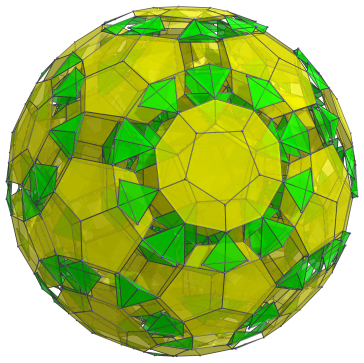For clarity, we've omitted all the pentagonal prisms and triangular prisms, showing only the dodecahedra and tetrahedra. We've also omitted cells that lie on the far side of the polytope.

This projection gives us a little glimpse into the structure of the runcinated 120-cell. We explore its structure in detail in the next section.

## Structure

The 120 dodecahedral cells of the runcinated 120-cell correspond with the facets of the 120-cell, and its 600 tetrahedral cells correspond with the facets of the 600-cell. Straddling them are 720 pentagonal prisms, corresponding with the faces of the 120-cell (or the edges of the 600-cell) and 1200 triangular prisms, corresponding with the edges of the 120-cell (or the faces of the 600-cell). Thus, as we shall see, the structures of both the 120-cell and the 600-cell are reflected in the runcinated 120-cell.

We shall explore the structure of the runcinated 120-cell by examining its parallel projection in 3D, centered on a dodecahedral cell. Like the other highly-symmetric polychora, the runcinated 120-cell approximates a 4D hypersphere; so to help us visualize the layout of its cells, we divide them into layers, each of which corresponds with a particular “latitude” or “band” on the hypersphere.

### First Layer

The first layer of cells consist of a single dodecahedron, the nearest cell to the 4D viewpoint. This dodecahedron is the “north pole” cell, which projects to the center of the 3D image, as shown below: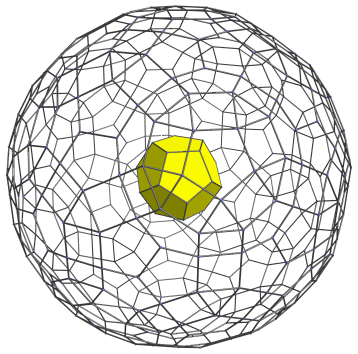For clarity, we've omitted all edges except those lying on the limb of the projection and the nearest dodecahedral cell.

### Second Layer

The pentagonal faces of the nearest dodecahedral cell are joined to 12 pentagonal prisms, which are shown in the next image: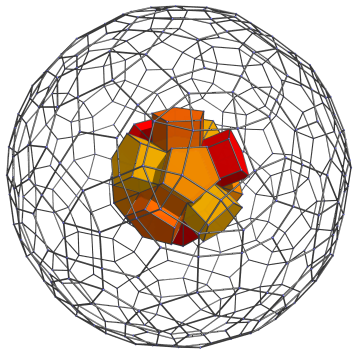Straddling these pentagonal prisms are triangular prisms that touch the original dodecahedron's edges. These are shown next:The remaining 20 gaps between these prisms are filled in by 20 tetrahedral cells, corresponding with the 20 tetrahedra surrounding a vertex in the 600-cell. They are shown in green below: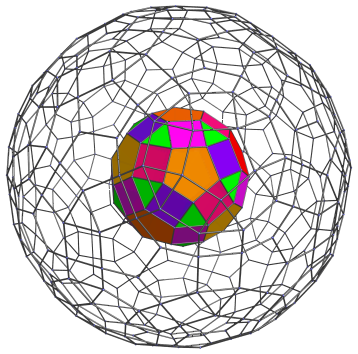The next image hides the prisms in the second layer, in order to show the relationship between the dodecahedron, which corresponds with the 120-cell, and the 20 tetrahedra, which correspond with the 600-cell: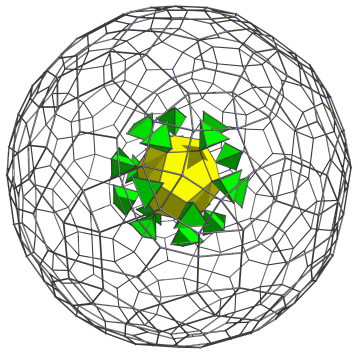As you can see, the triangular prisms that straddled the pentagonal prisms fit between these tetrahedra along the dodecahedron's edges.

### Third Layer

Building on the cells in the previous layer, we now add 30 pentagonal prisms which are joined to the 30 triangular prisms from the previous layer. These pentagonal prisms are shown below: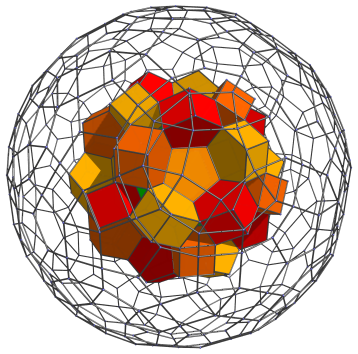Now it is obvious that each ring of 5 pentagonal prisms surrounds a depression which can be filled in by the next layer of dodecahedra. There are 12 such depressions, corresponding with the faces of the dodecahedron in the first layer. The next image shows these dodecahedra added: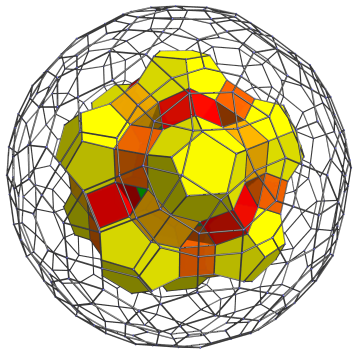Of course, we cannot neglect the triangular holes into which 20 triangular prisms can be fitted: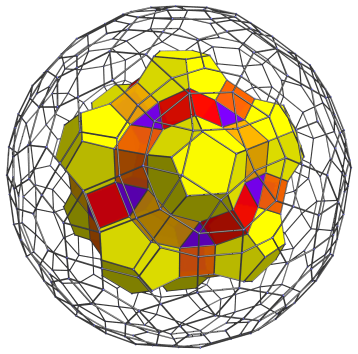### Fourth Layer

Atop the last 20 triangular prisms in the third layer are 20 more tetrahedra. These cells correspond with the second layer of tetrahedra in the 600-cell, the ones that share a face with the 20 “north pole” tetrahedra clustered around a single vertex in the 600-cell.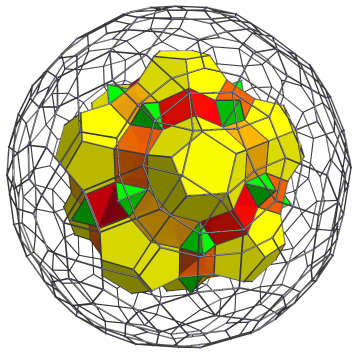These tetrahedra are joined to 60 triangular prisms that lie on the square faces of the pentagonal prisms. We add them below: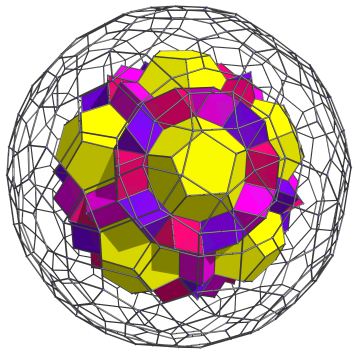Straddling these triangular prisms and the pentagonal faces of the yellow dodecahedra are 60 more pentagonal prisms, which are shown below: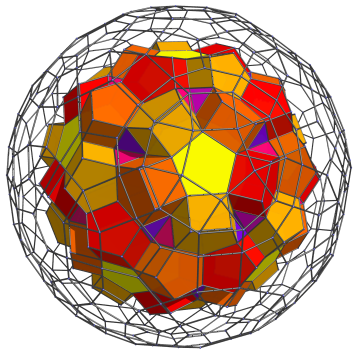Snuggled against the triangular faces of the triangular prisms now almost covered over are 30 tetrahedra, shown next in green: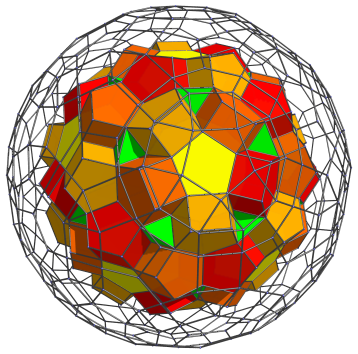### Fifth Layer

Of course, the 30 tetrahedra are joined to 60 more triangular prisms via their two exposed triangular faces. We add them now: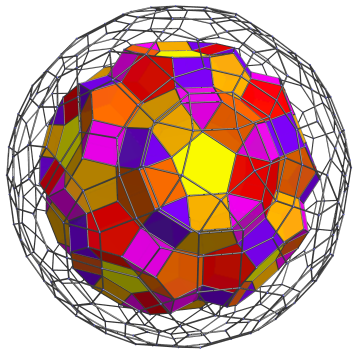Next, we add 42 pentagonal prisms: 12 attached to the exposed pentagonal faces of the yellow dodecahedra, and 30 straddling each pair of triangular prisms: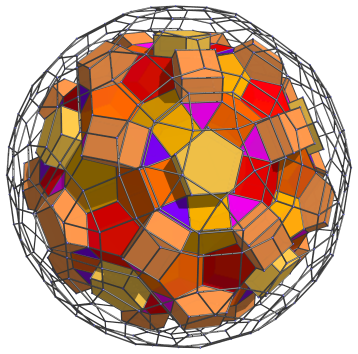Around each of the 12 pentagonal prisms that overlie the dodecahedra, there is a ring of 5 triangular prisms, making a total of another 60 triangular prisms: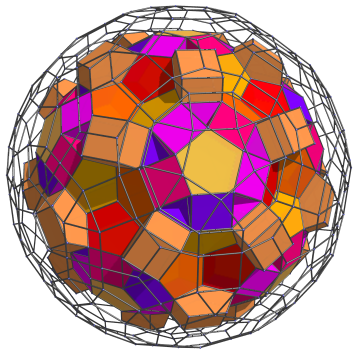### Sixth Layer

The next layer of cells begins with 20 dodecahedra that fit into the bowl-shaped depressions formed by pentagonal prisms seen in the previous layer: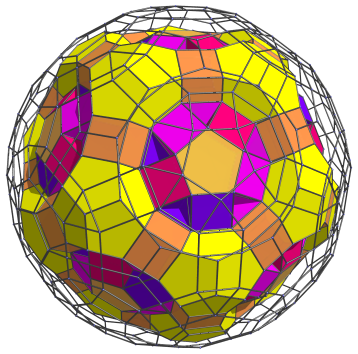The tetrahedron-shaped holes left in each ring of 5 dodecahedra, of course, are filled by tetrahedral cells, shown in green next: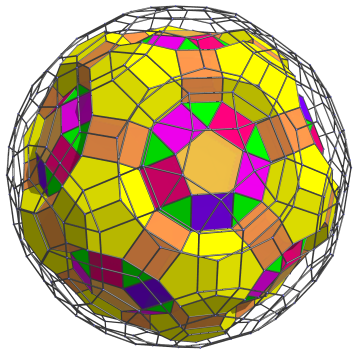There are 60 tetrahedra added in total.

The exposed square faces of the triangular prisms between each pair of the tetrahedra are joined to a pentagonal prism each. This makes another 60 pentagonal prisms: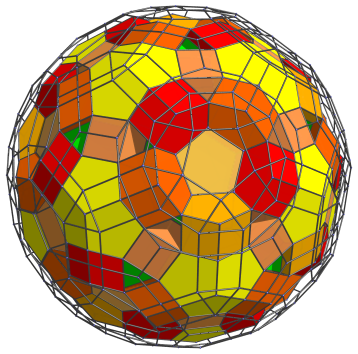### Seventh Layer

Those triangular holes visible from the previous layer are obviously where more triangular prisms can fit, 60 of them: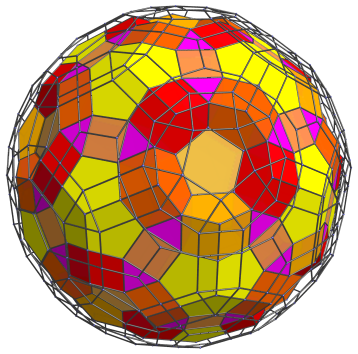And of course, the bowl-shaped depressions formed by the circles of 5 pentagonal prisms are where another 12 dodecahedra can fit: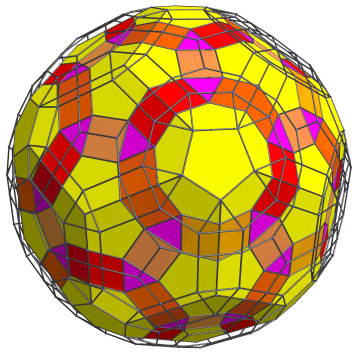### Eighth Layer

We're almost at the “equator” of the runcinated 120-cell. On the previous layer of cells there is now another layer of prisms and tetrahedra. We begin with 60 tetrahedra that sit atop the magenta triangular prisms added in the previous layer: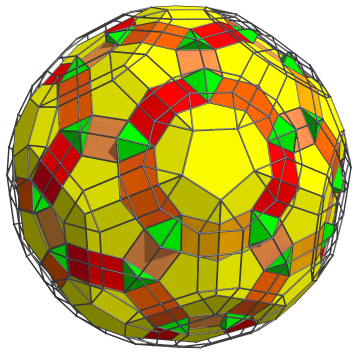Since we're now near the “equator”, the cells are seen at a sharp angle, so these tetrahedra appear quite flattened. This is the foreshortening caused by the projection; in 4D, they are perfectly regular tetrahedra.

Surrounding these tetrahedra are 3 triangular prisms each, with one of them shared with another tetrahedron.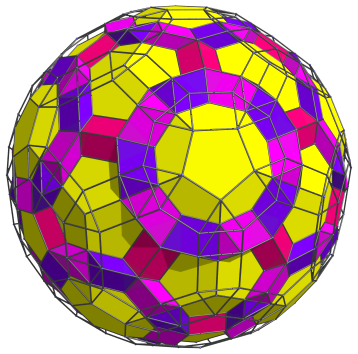There are a total of 150 triangular prisms added here. Straddling 120 of them are another 60 tetrahedra, shown below: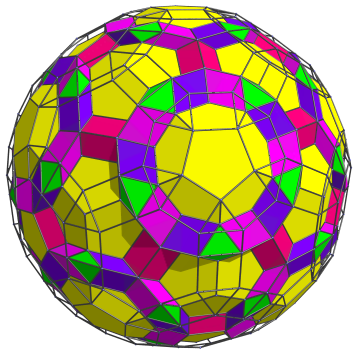### Ninth Layer

The exposed pentagonal faces of the yellow dodecahedra are joined to another layer of pentagonal prisms. The next image shows 60 of them: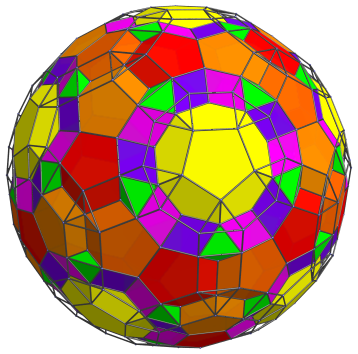The other 60 pentagonal prisms are added below: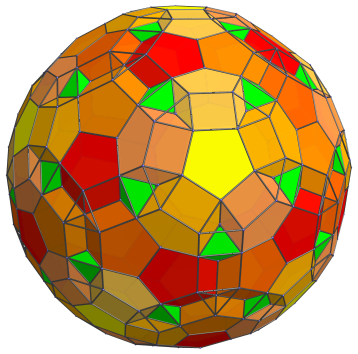These are the last of the pentagonal prisms before we reach the equator.

There are 20 more tetrahedra before the equator: these fit between circles of 3 pentagonal prisms in the first set of 60 pentagonal prisms.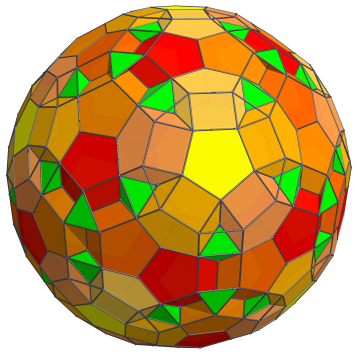Between these 20 tetrahedra and the other 60 visible ones, we can fit another 60 triangular prisms: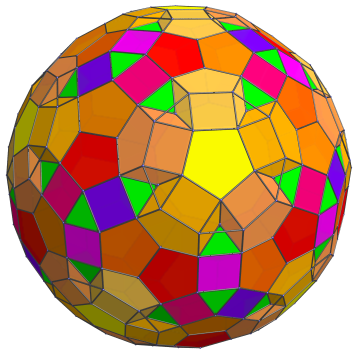The final gaps before the equator are filled in by the last set of 60 triangular prisms, as shown below: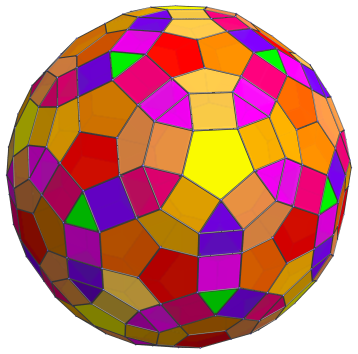### The Equator

Finally we reach the equator of the runcinated 120-cell. There are 30 dodecahedral cells at the equator, just like in the 120-cell. We display them below: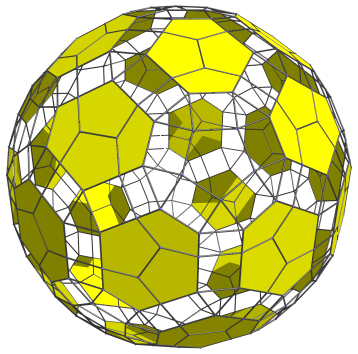To reduce visual clutter, we omit the cells from before, that do not lie on the equator. Since these cells are at a 90° angle from the 4D viewpoint, they are foreshortened into hexagons.

There are 60 tetrahedra that lie on the equator: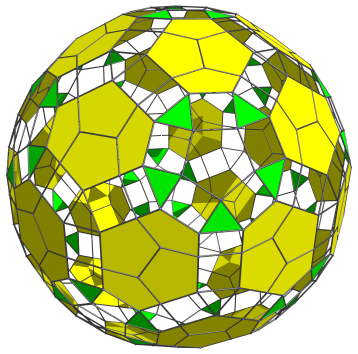Each of the 12 circles of 5 tetrahedra surround one of a set of 12 pentagonal prisms that lie on the equator. These pentagonal prisms are shown below: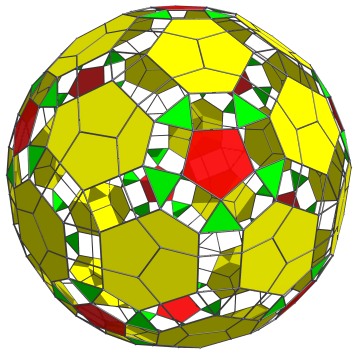These pentagonal prisms actually share an edge with each surrounding tetrahedron, but due to foreshortening, that edge has been collapsed into a point, and the pentagonal prisms have collapsed into pentagons.

There are actually 60 other pentagonal prisms that lie on the equator, though they are not easy to pick out because they lie sideways to the 4D viewpoint, causing them to appear as rectangles, as shown below in orange: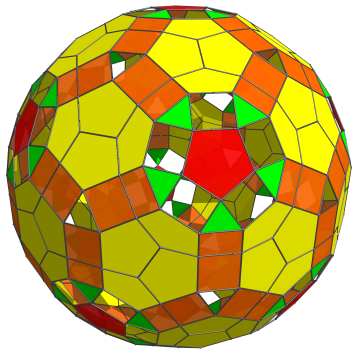Between each triplet of pentagonal prisms is a triangular prism foreshortened into a triangle. There are 20 of these, as seen below in magenta: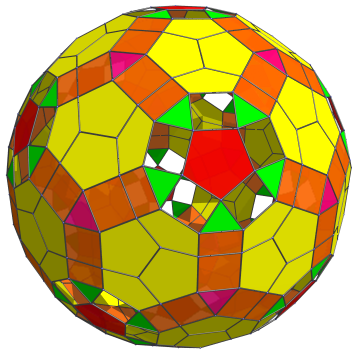Finally, the remaining rectangular gaps are filled in by the last 60 triangular prisms, shown next: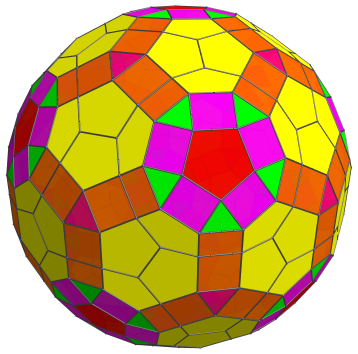This completes the equator of the runcinated 120-cell.

### The Southern Hemisphere

From this point on, the 9 layers in the “northern hemisphere” repeat themselves in reverse to form the “southern hemisphere”, which perfectly mirrors the northern hemisphere.

### Summary

The following table summarizes the cell counts in each of the layers of the runcinated 120-cell:

Region Layer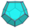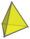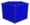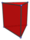Northern hemisphere 1 1 0 0 0
2 0 20 12 30
3 12 0 30 20
4 0 20 + 30 = 50 60 60
5 0 0 12 + 30 = 42 60 + 60 = 120
6 20 60 60 0
7 12 0 0 60
8 0 60 + 60 = 120 0 60/2 + 60*2 = 150
9 0 20 60 + 60 = 120 60 + 60 = 120
Subtotal 45 270 324 560
Equator 30 60 12 + 60 = 72 20 + 60 = 80
Southern hemisphere 9 0 20 120 120
8 0 120 0 150
7 12 0 0 60
6 20 60 60 0
5 0 0 42 120
4 0 50 60 60
3 12 0 30 20
2 0 20 12 30
1 1 0 0 0
Subtotal 45 270 324 560
Grand total 120 600 720 1200

## Animation

The dodecahedral cells of the runcinated 120-cell are exactly the dodecahedral cells of the 120-cell expanded outwards radially. As such, they are a useful method of visualizing the layout of cells in the 120-cell. The following animation shows these 120 dodecahedral cells rotating in 4D: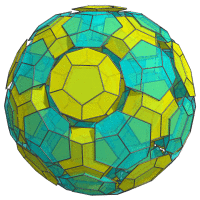The rotation is taking place in the plane where one of the runcinated 120-cell's great circles lie. The yellow cells lie on the near side, whereas the cyan cells lie on the far side. Some cells can been seen transitioning from the near side to the far side, and vice versa, as the polytope rotates. As they transition, they turn “inside-out” from our 3D viewpoint. There is a ring of blue cells (horizontal near the bottom of the projection) that are lie in the stationary plane of the rotation; these are rotating “in place”. This ring of cells is analogous to a rotational pole in 3D; in 4D it is not just a pole, but a ring.

## Coordinates

The Cartesian coordinates of the runcinated 120-cell, centered on the origin and having edge length 2, are all permutations of coordinates and changes of sign of:

 (1, 1, φ3, 3+4φ) (φ, φ, φ, 2+5φ) (φ, 2+φ, φ4, φ4) (φ2, φ2, 1+3φ, 3φ2) (φ2, 1+3φ, 1+3φ, 1+3φ) (φ3, φ3, φ3, 1+4φ)

along with the even permutations of coordinates and all changes of sign of:

 (0, 1, φ4, 3φ2) (0, 1, 2+5φ, φ2) (0, 2φ, 2φ3, 2φ2) (0, φ2, 1+4φ, φ4) (0, φ2, 3+4φ, 2+φ) (1, φ, 2φ3, 1+3φ) (1, 2φ2, 1+3φ, φ4) (φ, φ2, 2φ, 3+4φ) (φ, φ3, 3φ2, 2φ2) (φ2, 2+φ, φ3, 2φ3) (2φ, φ3, φ4, 1+3φ)

where φ=(1+√5)/2 is the Golden Ratio.

Last updated 02 Feb 2023.